Home Participants Programme Abstracts Online Talks PhotographsLondon Mathematical Society Durham Symposium
Operator Theory and Spectral Analysis
Tuesday 2nd August - Friday 12th August 2005

## List of abstracts

 Mikhail Agranovich (Moscow Institute of Electronics and Mathematics) Monday 8th August 09:20 Poincare-Steklov type operators in domains with Lipschitz boundaries We consider a strongly elliptic second order system in a bounded domain. The principal part of the system is written in a divergent form, but the system is not necessarily self-adjoint. The boundary conditions are mixed and contain the spectral parameter on a part of the boundary. Assuming that the domain is Lipschitz, we consider the problem in the variational setting by means of the classical variational approach and describe some spectral properties of the corresponding Neumann-to-Dirichlet type operators in the simplest Sobolev spaces. These operators admit more explicit representation if it is possible to use surface potentials, in particular if the coefficients in the system are constant. The information is more complete in the case of N-to-D operators on the whole boundary. In the general case, assuming additional smoothness, we use the Wiener-Hopf method. Similar approaches are used for exterior mixed problems. In addition, we consider transmission spectral problems in the case of a non-closed boundary. A lot of references will be given in the talk. Charles Batty (University of Oxford) Saturday 6th August 17:10 Resolvent conditions for perturbations of semigroup generators and solutions of delay equations There are well known results of the form that if \$A\$ generates a \$C_0\$-semigroup of a certain type and \$B\$ is a perturbation of a certain type then \$A+B\$ generates a \$C_0\$-semigroup. We give some converse results for generators of semigroups and related operators, and some results about differentiability of solutions of delay equations. Michael Berry (University of Bristol) Wednesday 10th August 11:50 Physics of nonhermitian degeneracies Decoherence makes quantum evolution nonunitary, and such systems, where some freedoms are ignored, can be described by nonhermitian hamiltonian operators. These differ most dramatically from hermitian operators in the neighbourhood of degeneracies. Several examples of non-hermitian degeneracy-dominated physical phenomena will be given, in laser physics, atom optics, and crystal optics. Albrecht Boettcher (TU Chemnitz) Monday 8th August 11:50 From Toeplitz eigenvalues to spectra of canonical higher-order ordinary differential operators The talk is concerned with a sequence of constants which appear in several problems. These problems include the minimal eigenvalue of certain positive definite Toeplitz matrices, the minimal eigenvalue of some higher-order ordinary differential operators, the norm of the Green kernels of these operators, the best constants in Wirtinger-Sobolev inequalities, and the conditioning of a special least squares problem. We give an introduction to all these topics, discuss the asymptotics of the sequence, and disclose the mystery of the appearance of some regular numbers in the spectra of certain differential operators. The talk is based on joint work with Harold Widom. Lyonell Boulton (Heriot-Watt University) Wednesday 3rd August 10:20 On the oscillation of eigenfunctions of fourth-order two-point boundary value problems. It is well known that the notion of conjugate points for linear systems of ordinary differential equations, allows us to characterise oscillation properties of solutions of boundary value problems of order greater than two. However, in the general case, a conjugate point need not be a place where the solution vanishes. In this talk we consider counting true zeros of solutions. Our main goal will be to discuss how to recover results in the spirit of the classical Sturm-Liouville theorem for fourth-order eigenvalue problems. Simon Chandler-Wilde (University of Reading) Thursday 4th August 17:10 Spectral problems associated with scattering by unbounded surfaces We consider the problem of scattering of an acoustic wave by an unbounded surface that is the graph of some bounded continuous function, f, and the reformulation of this problem as a second kind integral equation with an integral operator that depends on the function f as a parameter. We study how the spectrum depends on f and on the function space on which the operator acts, making connections with Floquet-Bloch theory in the case when f is periodic, and with recent theories of generalised convolution operators. Walter Craig (McMaster University) Thursday 4th August 11:50 Hamiltonian PDE Abstract: Many nonlinear evolution equations can be posed as Hamiltonian systems with infinitely many degrees of freedom. These include some of the central equations of mathematical physics; the nonlinear wave equation, the nonlinear Schroedinger equation, the Euler equations for free surfaces in fluid dynamics, and others. In a neighborhood of an equilibrium, the linearized equations are those of a harmonic oscillator and thus their solutions exhibit periodic, quasi-periodic and almost periodic motion. To construct solutions of the same nature for the nonlinear partial differential equations is a small divisor problem in general. This talk will give an overview of a variational principle which arises, and an overview of some of the analytic techniques and results from Hamiltonian dynamical systems applied to PDE, which have been developed to address this class of problems. Michael Dritschel (University of Newcastle) Saturday 6th August 10:20 Interpolation in semigroupoid algebras A seminal result of Agler characterises the so-called Schur-Agler class of functions on the polydisk in terms of a unitary colligation transfer function representation. We generalise this to the unit ball of the algebra of multipliers for a family of test functions/reproducing kernels over a broad class of semigroupoids. There is then an associated interpolation theorem. Besides leading to solutions of the familiar Nevanlinna-Pick and Carathéodory-Fejér interpolation problems and their multivariable commutative and noncommutative generalisations, this approach also allows us to consider more exotic problems, such as interpolation over domains where the Pick condition is not satisfied and interpolation over a variety of matrix algebras. Des Evans (Cardiff University) Friday 5th August 10:20 On the spectrum in Smilansky's model of irreversible quantum graphs: the 2-oscillator case (Joint work with M. Solomyak) In his talk M.Solomyak has already discussed Smilansky's model and described results established on the spectrum of the underlying self-adjoint operator when the system involves one harmonic oscillator. In this talk the analyic obstacles encountered in the case of two harmonic oscillators will be discussed and recent results obtained jointly with M. Solomyak given. Edward Fraenkel (University of Bath) Thursday 11th August 11:50 A constructive existence proof for the extreme Stokes wave A troublesome, non-linear integral equation is solved in the following naive way. First, an explicit formula defines an approximation with numerically small error; secondly, the Frechet derivative at this approximation is inverted; thirdly, inequalities are found for the non-linear remainder. There results a contraction map for the difference between the exact solution and the approximation. Leonid Friedlander (University of Arizona) Friday 5th August 15:50 The determinant of a differential operator on a metric graph We derive a formula for computing the determinant of an operator on a metric graph. In the case of the Laplacian, this formula is rather explicit. Alex Gamburd (Stanford University) Tuesday 9th August 10:20 Poisson-Dirichlet distribution for random Belyi surfaces Brooks and Makover introduced an approach to studying the global geometric quantities (in particular, the first eigenvalue of the Laplacian, injectivity radius and diameter) of a "typical" compact Riemann surface of large genus based on compactifying finite-area Riemann surfaces associated with random cubic graphs; by a theorem of Belyi these are "dense" in the space of compact Riemann surfaces. The question as to how these surfaces are distributed in the Teichmuller spaces depends on the study of oriented cycles in random cubic graphs with random orientation; Brooks and Makover conjectured that asymptotically normalized cycles lengths follow Poisson-Dirichlet distribution. We present a proof of this conjecture using representation theory of the symmetric group. Alastair Gillespie (University of Edinburgh) Friday 5th August 17:10 Weighted ergodic theory and dimension free estimates (Joint work with J.L. Torrea)I shall discuss a weighted ergodic theory associated with a strongly continuous one-paramenter group of positive operators acting on an Lp space which parallels the classical Ap theory. This is then used to obtain dimension free weighted inequalities for certain classical operators. Ilya Goldsheid (QMUL) Thursday 4th August 17:40 Random diffeomorphisms, Lyapunov exponents, and random operators I shall discuss recent results about contraction properties of random diffeomorphisms acting on skew product spaces. It turns out that these lead, in particule, to control of Lyapunov exponents of products of random matrices. The latter, in turn, are responsible for the spectral properties of random operators. Oleksandr Gomilko (Kiev National University of Trade and Economics) Thursday 11th August 17:40 The Caley transform of the generator of a uniformly bounded C_0-semigroup Gian Michele Graf (ETH-Zurich) Saturday 6th August 11:50 Equality of bulk and edge Hall conductances in a mobility gap (Joint work with A. Elgart, J. Schenker.)I will present two interpretations of the integer quantum Hall effect on the basis of either bulk or edge currents, introduce the corresponding conductances, and heuristically show that they are equal. In a more strict part, I will consider quantum Hall systems for which the Fermi energy falls in a band where bulk states are localized, this being the prerequisite for a Hall plateau. After defining the edge conductance in a manner appropriate to this situation, I will prove its equality to the bulk value. Rostyslav Hryniv (Institute for Applied Problems of Mechanics and Mathematics, NASU) Wednesday 3rd August 17:40 Inverse spectral problems for Bessel operators (Joint work with S Albeverio and YV Mykytyuk) Let q be a real-valued distribution from the space W-12(0,1) and let S denote the Bessel operator on L2(0,1) given by (Sy)(x) = - y"(x) + m(m-1)y(x)/x2 + q(x) y(x), where m is a nonnegative integer. We study the inverse spectral problem of reconstructing the operator S from two spectra corresponding to two different boundary conditions at the endpoint x=1. Serguei Iakovlev (Simon Bolivar) Poster Operators of Friedrichs with a non-trivial singular spectrum Ilia Kamotski (University of Bath) Monday 8th August 18:10 Structure of the spectrum of acoustic operator with singularly perturbed periodic coefficients It is well known that one-dimensional Schrodinger operator with periodic potential ``always" has band gaps in its spectrum. On the contrary, in the multi-dimensional case such gaps are extremely rare. On the other hand, their existence is of great importance, for example for the presence of localised states in photonic crystals. We consider 3D-homogenous acoustic media perturbed by the set of small periodically distributed inclusions with high compressibility. Previously known examples of gaps for high contrast materials are where the contrast is imposed either on thin films or on domains with asymptotically non zero volume. We investigate media with small defects localised in the neighborhood of isolated periodic set of points. This allows us to observe an explicit asymptotic mechanism for opening of the gaps. Using the method of matched asymptotic expansions we demonstrate the existence of gaps, derive their asymptotics and rigourously justify it. Further analysis of asymptotic representation of solutions shows that such media can be modelled by some operator with non-linear frequency dependence. This operator can be treated as an \$L_2\$ self-adjoint extension of the governing operator originally defined on functions equal to zero near the defects. Lev Kapitanski (University of Miami) Saturday 6th August 15:50 Geometry and analysis of the Faddeev model Thomas Kappeler (Universität Zürich) Friday 5th August 11:50 On geodesic exponential maps of the Virasoro group (Joint work with A. Constantin, B. Kolev, and P. Topalov) We study the geodesic exponential maps corresponding to Sobolev type right-invariant (weak) Riemannian metrics g(k) ( k = 0, 1, ...) on the Virasoro group Vir and show that for k = 2, 3, 4,... , but not for k = 0, 1, each of them defines a smooth Frechet chart of the unital element in Vir. The geodesic exponential map for k = 0 corresponds to the KdV equation and hence is not a local diffeomorphism near the origin. David Khmelnitskii (University of Cambridge) Wednesday 3rd August 17:10 Physicist's view on localisation The purpose of this talk is to present not rigorous prejudices and believes physics community has about localisation in different dimensions in magnetic field and without it. Boris Khoruzhenko (QMUL) Monday 8th August 10:20 Moments of spectral determinants of complex random matrices (Joint work with Y.V. Fyodorov) Let A be a fixed nxn matrix and U be a unitary matrix picked up at random from the unitary group U(n). We express the integer moments of the spectral determinant |\det(zI_n-AU)|^2 in terms of the characteristic polynomial of the matrix AA^*. This result provides a useful tool for studying the eigenvalue distributions of complex random matrices. Links between this problem, Kaneko's generalization of the Selberg integral and Zirnbauer's color-flavor transformation will be discussed. Anna Kirpichnikova (Loughborough University) Poster Inverse Boundary Spectral Problem for a Riemannian Polyhedron Vladimir Kisil (University of Leeds) Thursday 4th August 10:20 Algebraic and analytic aspects of covariant functional calculus Covariant functional calculus is defined as an intertwining operator between certain group representations [1, 2]. The intertwining property replaces the algebraic homomorphism required by the standard definition of functional calculus. This gives advantages in consideration of non-normal operators  and enable functional calculus of several non-commuting operators [1, 3]. Spectrum of operator (or a n-tuple of operator) is defined as the support of the functional calculus , i.e. collection of nonvoid intertwining operators with primary representations. The previous results [2, 3] was mainly dealing with finite-dimensional operators and thus have essentially algebraic nature. The passage to infinite dimensional operators highlights interesting analytic structure in the category of intertwining operators.  V.V. Kisil, Mobius transformations and monogenic functional calculus, Electron. Res. Announc. Amer. Math. Soc. 2 (1996), no. 1, 2633, (electronic) MR 98a:47018.  V.V. Kisil, Spectrum as the support of functional calculus, Functional Analysis and its Applications (Proceedings of the S. Banach Conference) (North-Holland) (V. Kadets and W. Zelazko, eds.), Math. Studies series, vol. 197, Elsevier Science Publishers, 2004, E-print: arXiv:math.FA/0208249, pp. 133142.  V.V. Kisil, Monogenic calculus as an intertwining operator, Bull. Belg. Math. Soc. Simon Stevin 11 (2005), E-print: arXiv:math.FA/0311285. Frédéric Klopp (Paris Nord) Tuesday 9th August 11:50 Resonances for slowly varying perturbations of a periodic Schrödinger operator In this talk, we present recent results on the width of the resonances for a slowly varying perturbation of a one-dimensional periodic operator. It is based on joint work with M. Marx. The perturbation is assumed to be analytic and local in the sense that it tends to a constant at infinity; the constants at both infinities may differ. Modulo an assumption on the relative position of the range of the local perturbation with respect to the spectrum of the background periodic operator, we show that the width of the resonances is essentially given by a tunneling effect in a suitable phase space. Vladimir Kozlov (Linköpings Universitet) Thursday 4th August 15:50 L2-perturbations of leading coefficients of elliptic operators: asymptotics of eigenvalues and similar problems Pavel Kurasov (Lunds Universitet) Thursday 11th August 17:10 Wigner-von Neumann perturbations of periodic Schrödinger operator (Joint work with S. Naboko Wigner-von Neumann type perturbations of the one-dimensional Schrödinger operator are considered. The asymptotics of the solution to the generalized eigenfunction equation is investigated. It is proven that the subordinated solution and therefore an embedded eigenvalue may occur at the points on the continuous spectrum satisfying a certain resonance (quantization) condition between the frequencies of the perturbation, the frequency of the potential and the corresponding quasimomentum. Ari Laptev (KTH, Stockholm) Monday 8th August 15:50 Spectral inequalities for many particles We consider a number of inequalities for multi-particle Schrödinger operators. Optimal constants appearing in such inequalities are related to some elementary problems from geometrical combinatorics. Michael Levitin (Heriot-Watt University) Thursday 11th August 10:20 Embedded eigenvalues of the Laplacian for domains with cylindrical ends (Joint work with M Marletta) In a work motivated by a wide class of waveguide-type problems, we give some upper estimates on a number of eigenvalues embedded into the essential spectrum of a Laplacian acting on a domain with cylindrical outlets to infinity. We also construct a simple algorithm for finding such eigenvalues numerically. The algorithm also applies to finding complex resonances, and allows an easy modification for other differential operators and infinite geometries. Marko Lindner (TU Chemnitz) Friday 5th August 10:50 Generalized collective compactness and limit operators Vitali Liskevich (University of Bristol) Monday 8th August 10:50 Gaussian bounds for propagators perturbed by potentials (Joint work with H. Vogt and J.Voigt) We develop the perturbation theory for propagators, with the objective to prove Gaussian bounds. Let U be a strongly continuous propagator, i.e., a family of operators describing the solutions of a non-autonomous evolution equation, on an Lp-space, and assume that U is positive and satisfies Gaussian upper and lower bounds. Let V be a (time-dependent) potential satisfying certain Miyadera conditions with respect to U. We show that then the perturbed propagator enjoys Gaussian upper and lower bounds as well. Annemarie Luger (Vienna University of Technology) Poster On eigenvalues of self-adjoint extensions (Joint work with Jussi Behrndt, TU Berlin) Let S be a symmetric operator (or relation) with finite defect index in some space K and let A be a self-adjoint extension of S, which acts in a larger space K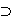K. Often (eg. if K is a Hilbert space) the compressed resolvent PK(A-z)-1|K is then given like in Krein's formula. In particular there appears a parameter T(z) and m(z), the corresponding Q-function of a pair (A0,S) for some fixed self-adjoint extension A0 in K. It turns out that in this situation - under some additional assumption on the parameter T - the eigenvalues of A are given as the generalized zeros of the function m(z)+T(z). Here the inner product on the space K need not be positive definite, however, locally we assume the existence of only finitely many negative squares. Furthermore we point out the connection to an abstract boundary value problem. Marco Marletta (Cardiff University) Friday 5th August 17:40 Weak stability for an inverse Sturm-Liouville problem with finite spectral data and complex potential We consider a stability result for the Sturm-Liouville equation with complex potential in which the first N Dirichlet-Dirichlet eigenvalues and the first N Dirichlet-Neumann eigenvalues are known to within a given error. Although the problem is not uniquely solvable we show that all the complex potentials which solve it are close in a suitable weak norm. This is joint work with Rudi Weikard of University of Alabama at Birmingham. Yuri Netrusov (University of Bristol) Saturday 6th August 17:40 Sharp version of the Birman-Solomyak theorem on estimates of entropy numbers Nikolai Nikolski (Université Bordeaux) Wednesday 3rd August 11:50 Van der Waerden's problem and analytic capacities We discuss condition numbers of nxn matrices with a given lower bound of eigenvalues as n tends to infinity. The techniques used are zero sets capacities of finite sets with respect to the ususal holomorhic spaces, like some Besov spaces, or Beurling-Sobolev spaces. Leonid Parnovski (University College London) Tuesday 9th August 17:40 Distribution of lattice points in Euclidean and hyperbolic spaces (Joint work with R.Hill)We study the number of lattice points inside a ball (or an annulus) of large radius as a function of the centre of a ball. The average value of this function is the volume of the ball, and we obtain upper and lower bounds (and asymptotics in some cases) for the average deviation of this function from its average as well as for its variance. Jonathan Partington (University of Leeds) Tuesday 9th August 10:50 The resolvent test for admissibility of semigroups and Volterra equations (Joint work with Birgit Jacob) Consider the system [dx(t)/dt]=Ax(t), y(t)=Cx(t), for t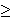0, where each x(t) lies in a fixed Hilbert space H. Here the operator A with domain D(A)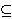H generates a contraction semigroup, and C:D(A)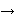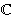n is a finite-rank observation operator. The property of admissibility, || y (.)||L2(0,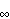)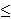const. || x(0) ||, is known to be related (by the Weiss conjecture) to the growth of || C(sI-A)-1 || for s in the right half-plane: this theory extends certain classical results on Hankel operators and Carleson embeddings. We review these ideas, and provide an appropriate generalization to Volterra systems, as well as a counterexample to the Weiss conjecture in this context. David Pearson (University of Hull) Thursday 4th August 10:50 Spectral density for one-dimensional Schrodinger operators For various classes of potential q, there is a well established link between spectral density of the corresponding Schrodinger operator on the half line, subject to boundary condition at x=0, and the large x asymptotics of quadratic forms in solutions of the Schrodinger equation and their derivatives. Original results of this kind are due to Titchmarsh in the case of potentials of L1 class. This talk will present a general theory of quadratic forms in solutions of the Schrodinger equation, and show how the theory may be used to determine spectral density for wide classes of potential, including in particular slowly decreasing potentials, periodic potentials, and examples of Wigner-von Neumann type. The theory will also be applied to the determination of spectral density for problems with two singular endpoints. Some of the results obtained have been implemented numerically in calculations of spectral density, in collaboration with Charles Fulton and Steve Pruess. Beatrice Pelloni (University of Reading) Saturday 6th August 10:50 The spectral analysis of linear differential operators - a new approach Michael Plum (Universität Karlsruhe) Thursday 4th August 09:20 An existence and enclosure method for nonlinear elliptic boundary value problems The lecture will be concerned with numerical enclosure methods for nonlinear elliptic boundary value problems. Here, analytical and numerical methods are combined to prove rigorously the existence of a solution in some "close" neighbourhood of an approximate solution computed by numerical means. Thus, besides the existence proof, verified bounds for the error (i.e. the difference between exact and approximate solution) are provided. For the first step, consisting of the computation of an approximate solution in some appropriate Sobolev space, no error control is needed, so a wide range of well-established numerical methods (including multigrid schemes) is at hand here. Using this approximate solution, the given problem is written as a fixed-point equation for the error, and the goal is to apply a fixed-point theorem providing the desired error bound. The conditions required by the chosen fixed-point theorem (e.g., compactness or contractivity, inclusion properties for a suitable subset etc.) are now verified by a combination of analytical arguments (e.g., explicit Sobolev embeddings, variational characterizations etc.) and verified computations of certain auxiliary terms, in particular of eigenvalue bounds for the linearization of the given problem at the approximate solution. The method is illustrated by several examples (on bounded as well as on unbounded domains), where in particular it gives existence proofs in cases where no purely analytical proof is known. Iosif Polterovich (Université de Montréal) Wednesday 10th August 10:20 The spectral function and the remainder in local Weyl's law: View from below The talk focuses on asymptotic lower bounds for the spectral function of the Laplacian and for the pointwise error term in Weyl's law on manifolds. I will first discuss some general results and then explain how the techniques of thermodynamic formalism for hyperbolic flows yield stronger estimates in the negatively curved case. This is a joint work with Dmitry Jakobson (McGill). Sandra Pott (University of Glasgow) Thursday 11th August 10:50 Admissibility and exact observability of observation operators We discuss some new criteria for admissibility and exact observability of observation operators on semigroups. (This is joint work with J. Partington.) Roman Romanov (St Petersburg State University) Poster Spectral singularities and asymptotics of contractive semigroups Joint work with Sergey NabokoThe problem of localization of spectral singularities of dissipative operators in terms of the asymptotic of the corresponding exponential function is studied. We give a solution to this problem for the singularities of higher orders in the frame of the perturbation theory. Grigori Rozenblioum (Chalmers University of Technology) Tuesday 9th August 09:20 On eigenvalues of the Pauli and magnetic Schrödinger operators with variable magnetic field: zero modes and splitting of Landau levels. Michael Ruzhansky (Imperial College London) Thursday 4th August 18:10 Limiting absorption principle in the critical case and applications In general, the limiting absorption principle allows one to deal with resolvents of operators near the spectrum by taking limits in weighted Sobolev spaces. We will talk about the critical cases of this where one observes the propagation of singularities along trajectories for the corresponding evolution equation. Results will be applied to smoothing problems for Schrodinger equation and to nonlinear equations with null-form structure. The talk is based on the joint work with Mitsuru Sugimoto (Osaka). Yuri Safarov (King's College London) Poster Multidimensional numerical range Eugene Shargorodsky (King's College London) Saturday 6th August 18:10 The Riemann-Hilbert problem with a vanishing coefficient that arises in nonlinear hydrodynamics The talk, based on joint works with J.F. Toland and with J. Virtanen, will describe various (non)uniqueness results for the Riemann-Hilbert problem in the case when the coefficient is continuous and is allowed to vanish on an arbitrary closed set of measure zero. In most earlier works the zero set of the coefficient was finite and the coefficient had a simple power- or power-log-like behaviour in a neighbourhood of its zeros. The motivation for the problems we consider comes from the theory of Bernoulli free-boundary problems and, in particular, from the variational theory of Stokes waves. Stanislav Shkarin (King's College London) Friday 5th August 18:10 On possible spectral structure of linear continuous operators For a class X of topological vector spaces let P(X) (resp. Q(X)) be the set of triples (A,B,C) of subsets of the complex plane for which there exist E in X and a continuous (resp. closed densely defined) linear operator T acting on E, for which A is the point spectrum B is the continuous spectrum and C is the residual spectrum. While it is usually relatively easy to find necessary conditions for a triple (A,B,C) to belong P(X), the task to show that this conditions are sufficient, is much more complicated. It can be considered as a problem of spectral syntesis: for a given triple of sets (A,B,C), satisfying certain conditions to find a space E in X and an operator T acting on E such that A, B and C are exactly the parts of the spactrum of T. We characterize the sets P(Xn) and Q(Xn), n=1,2,3,4, where X1 is the class of separable Hilbert spaces, X2 is the class of separable reflexive Banach spaces, X3 is the class of separable Banach spaces and X4 is the class of separable Frechet spaces. For instance, the set P(X1)=P(X2) is exactly the set of triples (A,B,C) of subsets of complex plane for which A is a countable union of closed sets, B is a countable intersection of open sets and the union of A, B and C is non-empty and compact. The characterizations of P(X3) and P(X4) are given in terms of Souslin and co-Souslin sets. Mikhail Shubin (Northeastern University) Wednesday 3rd August 15:50 Can one see the fundamental frequency of a drum? (Joint work with Vladimir Maz'ya) In 1966 Marc Kac asked the famous question ``Can one hear the shape of a drum?", formulating a goal to determine the shape of a domain in Rn by its frequencies, or, equivalently, the eigenvalues of the Laplacian with the Dirichlet boundary conditions in this domain. This question stimulated thousands of papers in inverse spectral problems, which have numerous applications. The question in the title of this talk is somewhat opposite but seems very important too. The fundamental frequency of a domain corespponds to the lowest eigenvalue of the Dirichlet Laplacian in this domain. The goal is to find visual characteristics of the domain which determine or at least estimate the lowest eigenvalue. I will explain two-sided estimates for this eigenvalue in terms of a geometric characteristic of the domain which is defined with the help of the Wiener capacity. The results are joint work by V.Maz'ya and M.Shubin. They are closely related to a recent solution of a problem which was formulated by I.M.Gelfand in 1953 and solved in a recent paper by these authors (to appear in Annals of Mathematics). Valery Smyshlyaev (University of Bath) Tuesday 9th August 17:10 Spectral problems for high contrast periodic media and homogenisation Bands and gaps in the Bloch spectrum of an elliptic operator with highly contrasting coefficients can be described asymptotically explicitly (e.g. Hempel & Lienau; Zhikov). For "large defects" in such media (a problem related to wave localisation in photonic crystal fibres) we find an asymptotically complete set of eigenvalues and eigenfunctions inside the gap and prove the error bounds, using two-scale asymptotic analysis supplemented by high contrast homogenisation methods recently developed by V.V. Zhikov. We finally discuss a problem of resonances near the real axis in "finitely periodic" media with defects. Joint work with I.V. Kamotski. Zeev Sobol (Swansea) Monday 8th August 17:10 Dirichlet spaces with no reference measure. Nowdays Dirichlet form theory, started by Beurling and Deny and developed by Fukushima, heavily relyes on the L2 space of a reference measure. As shown by Fukushima, a change of the measure corresponds to a random time change of the process. Hence the attempt to develop a Dirichlet forms theory independent of the reference measure, and its counterpart - Markov processes theory independent of time change. Among the results, transience and reccurence, reducibility and explosion are shown independent of the measure choice. Michail Solomyak (Weizmann Institute) Friday 5th August 09:20 On the spectrum in Smilansky's model of irreversible quantum graphs In the model suggested by Smilansky one studies the interaction between a quantum graph and a system of one-dimensional harmonic oscillators attached at different points in the graph. Mathematically, the problem reduces to study of a family of partial differential operators, depending on several real parameters whose number is equal to the number of the oscillators attached. All these operators are defined by the same differential equation but different boundary conditions, of transmission type. Each condition involves a real parameter The family exhibits some interesting properties, including the phase transition at special values of the parameters. In the talk I suppose to describe the basic results for the leading case of one oscillator attached. Part of the results was obtained in cooperation with S.N.Naboko. Some results for several oscillators, obtained in cooperation with W.D.Evans, will be reported in his talk. Toshikazu Sunada (Meiji University) Wednesday 3rd August 09:20 Geometric theory of lattice vibrations This talk discusses, from a geometric standpoint, a classical subject in the solid state physics which dates back to Einstein's pioneering work in 1907 and its refinement by Debye in 1912 on the specific heat of a solid. The idea and method are taken up from discrete geometric analysis which has been recently developed for the spectral geometry of crystal lattices. This is the joint work with M. Shubin. Peter Topalov (Universität Zürich) Wednesday 3rd August 10:50 An inverse spectral result for a class of integrable billiard tables (Joint work with Georgy Popov) We consider a class of Riemannian manifolds with boundary having integrable geodesic flows and solve an inverse spectral problem involving the reconstruction of a smooth function defined on the boundary from the spectrum of the Laplace-Beltrami operator with Robin boundary conditions. Francoise Truc (Université Joseph Fourier) Monday 8th August 17:40 Accuracy on eigenvalues for Schrödinger Operator with a degenerate potential in the semi-classical limit (Joint work with Abderemane Morame, Université de Nantes, France) We consider a semi-classical Schrödinger operator -h^2 Delta + V with a degenerate potential V(x,y)=f(x)g(y); g is assumed to be a homogeneous positive function of m variables , smooth outside 0, and f is a smooth and strictly positive function of n variables with a strict convex minimum . In the case where f tends to infinity to infinity, the potential is still degenerate in the sense that it does not go to infinity at infinity; but the operator has compact resolvent and we give the asymptotic behaviour, for small values of the parameter h, of the number of eigenvalues less than a fixed energy. Without assumption on the limit of f, we give a sharp asymptotic behaviour of low eigenvalues bounded by some power of the parameter h, by improving Born-Oppenheimer approximation. Nikolai Vasilevski (CINVESTAV) Tuesday 9th August 15:50 Commutative algebras of Toeplitz operators and Berezin quantization We give a complete description of commutative C*-algebras of Toeplitz operators in terms of pencils of hyperbolic geodesics on the unit disk. We discuss as well the spectral behaviour of Toeplitz operators when the wight parameter of the Bergman space varies. Juan Weisz (Instituto de Desarollo Tecnologica para la Industria Química) Poster Systems of Commutating Matrices Dimitri Yafaev (Université de Rennes 1) Thursday 11th August 15:50 A particle in an inhomogeneous magnetic field. Classical and quantum equations of motion in the magnetic field of an infinite straight wire are explicitly solved. Boguslaw Zegarlinski (Imperial College London) Wednesday 10th August 10:50 Coercive inequalities and nonlinear parabolic problems in infinite dimensions We will present results on existence, uniqueness, hypercontractivity and ergodicity of nonlinear semigroups on infinite dimensional spaces in situations where coercive inequalities of Log-Sobolev type are available.